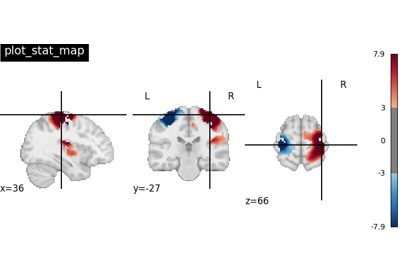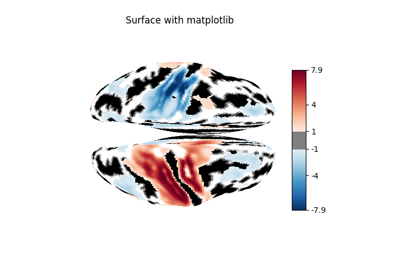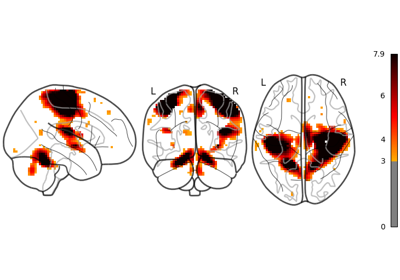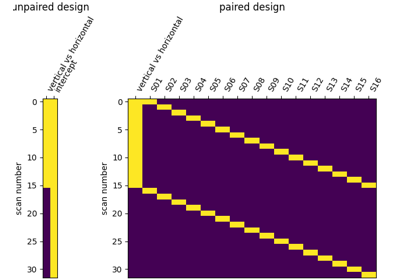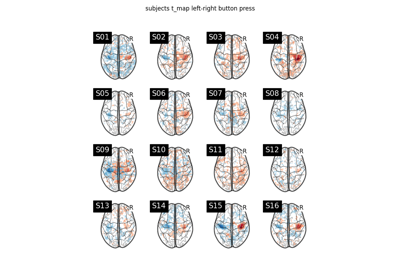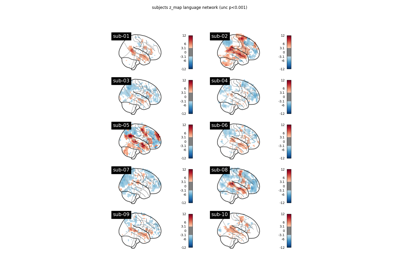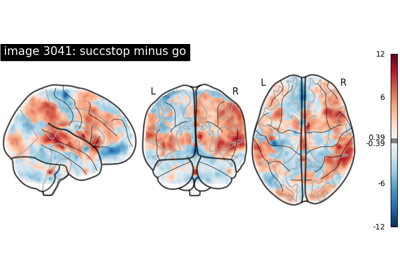Note

This page is a reference documentation. It only explains the function signature, and not how to use it. Please refer to the user guide for the big picture.

# 8.11.11. nilearn.plotting.plot_glass_brain¶

nilearn.plotting.plot_glass_brain(stat_map_img, output_file=None, display_mode='ortho', colorbar=False, cbar_tick_format='%.2g', figure=None, axes=None, title=None, threshold='auto', annotate=True, black_bg=False, cmap=None, alpha=0.7, vmin=None, vmax=None, plot_abs=True, symmetric_cbar='auto', resampling_interpolation='continuous', **kwargs)[source]

Plot 2d projections of an ROI/mask image (by default 3 projections: Frontal, Axial, and Lateral). The brain glass schematics are added on top of the image.

The plotted image should be in MNI space for this function to work properly.

Only glass brain can be plotted by switching stat_map_img to None.

Parameters
stat_map_imgNiimg-like object

See http://nilearn.github.io/manipulating_images/input_output.html The statistical map image. It needs to be in MNI space in order to align with the brain schematics.

output_file`str`, or None, optional

The name of an image file to export the plot to. Valid extensions are .png, .pdf, .svg. If `output_file` is not None, the plot is saved to a file, and the display is closed.

display_modestring, optional

Choose the direction of the cuts: ‘x’ - sagittal, ‘y’ - coronal, ‘z’ - axial, ‘l’ - sagittal left hemisphere only, ‘r’ - sagittal right hemisphere only, ‘ortho’ - three cuts are performed in orthogonal directions. Possible values are: ‘ortho’, ‘x’, ‘y’, ‘z’, ‘xz’, ‘yx’, ‘yz’, ‘l’, ‘r’, ‘lr’, ‘lzr’, ‘lyr’, ‘lzry’, ‘lyrz’. Default=’ortho’.

colorbar`bool`, optional

If `True`, display a colorbar on the right of the plots. Default=False.

cbar_tick_format: str, optional

Controls how to format the tick labels of the colorbar. Ex: use “%i” to display as integers. Default is ‘%.2g’ for scientific notation.

figure`int`, or `matplotlib.figure.Figure`, or None, optional

Matplotlib figure used or its number. If `None` is given, a new figure is created.

axes`matplotlib.axes.Axes`, or 4 tupleof `float`: (xmin, ymin, width, height), optional

The axes, or the coordinates, in matplotlib figure space, of the axes used to display the plot. If `None`, the complete figure is used.

title`str`, or None, optional

The title displayed on the figure. Default=None.

thresholda number, None, or ‘auto’, optional

If `None` is given, the image is not thresholded. If a number is given, it is used to threshold the image: values below the threshold (in absolute value) are plotted as transparent. If ‘auto’ is given, the threshold is determined magically by analysis of the image. Default=’auto’.

annotate`bool`, optional

If `annotate` is `True`, positions and left/right annotation are added to the plot. Default=True.

black_bg`bool`, or ‘auto’, optional

If `True`, the background of the image is set to be black. If you wish to save figures with a black background, you will need to pass facecolor=’k’, edgecolor=’k’ to `matplotlib.pyplot.savefig`. Default=False.

cmap`matplotlib.colors.Colormap`, or `str`, optional

The colormap to use. Either a string which is a name of a matplotlib colormap, or a matplotlib colormap object. Default=None.

alphafloat between 0 and 1, optional

Alpha transparency for the brain schematics. Default=0.7.

vmin`float`, optional

Lower bound of the colormap. If `None`, the min of the image is used. Passed to `matplotlib.pyplot.imshow`.

vmax`float`, optional

Upper bound of the colormap. If `None`, the max of the image is used. Passed to `matplotlib.pyplot.imshow`.

plot_absboolean, optional

If set to True (default) maximum intensity projection of the absolute value will be used (rendering positive and negative values in the same manner). If set to false the sign of the maximum intensity will be represented with different colors. See http://nilearn.github.io/auto_examples/01_plotting/plot_demo_glass_brain_extensive.html for examples. Default=True.

symmetric_cbar`bool`, or ‘auto’, optional

Specifies whether the colorbar should range from `-vmax` to `vmax` or from `vmin` to `vmax`. Setting to ‘auto’ will select the latter if the range of the whole image is either positive or negative.

Note

The colormap will always range from `-vmax` to `vmax`.

Default=’auto’.

resampling_interpolation`str`, optional

Interpolation to use when resampling the image to the destination space. Can be:

• “continuous”: use 3rd-order spline interpolation

• “nearest”: use nearest-neighbor mapping.

Note

“nearest” is faster but can be noisier in some cases.

Default=’continuous’.

Notes

Arrays should be passed in numpy convention: (x, y, z) ordered.

## 8.11.11.1. Examples using `nilearn.plotting.plot_glass_brain`¶